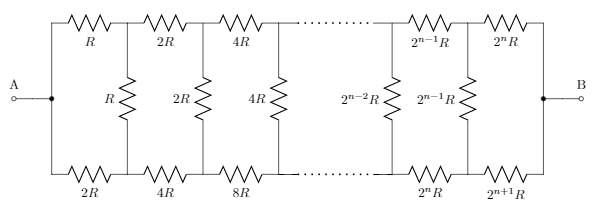# Bridge of resistors!There are $n + 1$ resistors in the upper and lower rows, respectively, and $n$ resistors between the rows.

The value of the total resistance between A and B can be represented as $(a \times 2^n - b)R$, where $a$ and $b$ are positive constants.

Find $a + b$.

×